# Pandas的下载

pandas下载成功后，会出现上述界面

pandas提供了使我们能够快速便捷地处理结构化数据的大量数据结构和函数

pandas兼具NumPy高性能的数组计算功能以及电子表格和关系型数据库灵活的数据处理功能

# Series

Series是一种类似于一维数组的对象，由数据(各种NumPy数据 类型)以及与之相关的数据标签(即索引)组成

``````import pandas as pd

data = [1, 2, 3]
series1 = pd.Series(data=data)
print(series1, type(series1))
# 获取下标为0的数据
print(series1)
print(">" * 70)
# 通过字典创建series
data1 = {1: "java", 2: "python", 3: "scala"}
series2 = pd.Series(data=data1)
print(series2, type(series2))
# 根据key值进行获取数据
print("根据key值获取数据:"+series2)
print(">" * 70)
# 创建series给定下标 根据给定key值进行创建
series3=pd.Series(data=data1, index=[2, 3])
print(series3)
``````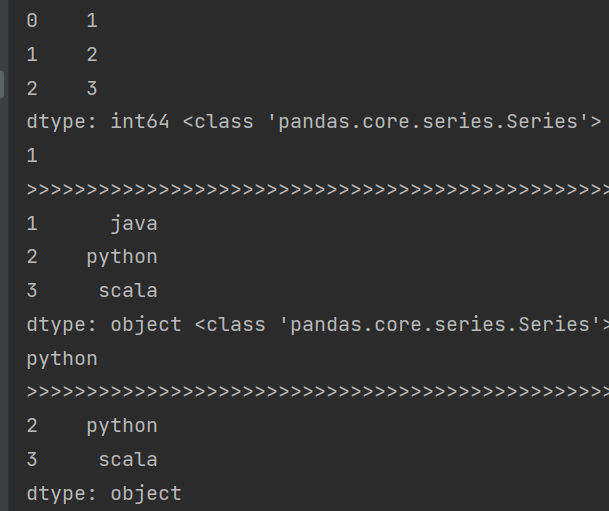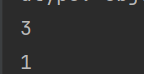# DataFrame

DataFrame是一个表格型的数据结构，它含有一组有序的列，每 列可以是不同的值类型(数值、字符串、布尔值等)

DataFrame既有行索引也有列索引，可以看做由Series组成的字典

## DataFrame的创建

DataFrame的创建可以通过字典创建，也可以通过列表创建，在使用列表创建时，默认情况下输出的列名称为数字顺序，可以在创建时加入设置列名称：columns=... ...

``````# 创建dataframe,通过字典创建
data2 = {
"Language": ["Python", "java", "scala", "sql"],
}
df = pd.DataFrame(data=data2)
print(df)
# 通过列表创建,可以增加列名称
data3 = [["java", "1"], ["Python", "2"], ["scala", "3"]]
df2 = pd.DataFrame(data=data3, columns=["Language", "Number"])
print(df2)
``````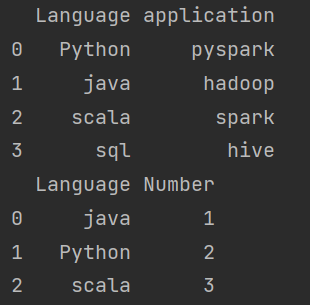``````data4=[,,]
df3=pd.DataFrame(data4)
print(df3,type(df3))
``````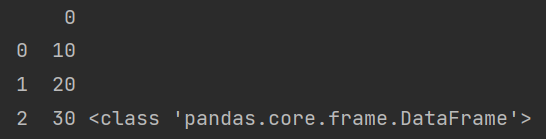## 删除操作：

``````# 删除df2中的列数据Number
df4=df2.drop(labels=["Number"], axis=1)
print(df4)
# 这里删除后的数据存到了df4中，df2中并未被删除
#使用inplace使df2中数据也被删除
print(">" * 70)
df2.drop(labels=["Number"], axis=1, inplace=True)
print(df2)
``````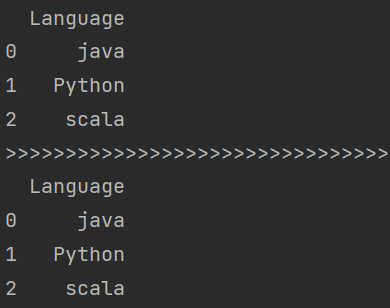## 获取操作：

dataframe中进行获取数据时，有多种获取数据的方法

1、通过key（列）获取数据

2、通过.+列名称进行获取

### 获取行数据：

1、通过dataframe.loc[index(行下标)]进行获取固定下标的一行数据

3、通过dataframe.tail()进行从后获取5行的数据

``````# 获取数据,通过key(列)进行获取
print(">" * 70)
print(df["application"], type(df["application"]))
# 通过.列名称获取
print(df.application,type(df.application))

data5 = {
"Language": ["A", "B", "C", "D", "E", "F", "G"],
"words": ["a", "b", "c", "d", "e", "f", "g"]
}
df5 = pd.DataFrame(data5)
print(df5.loc)  # 获取下标为2的数据
print(df5.tail()) # 获取后5行数据
``````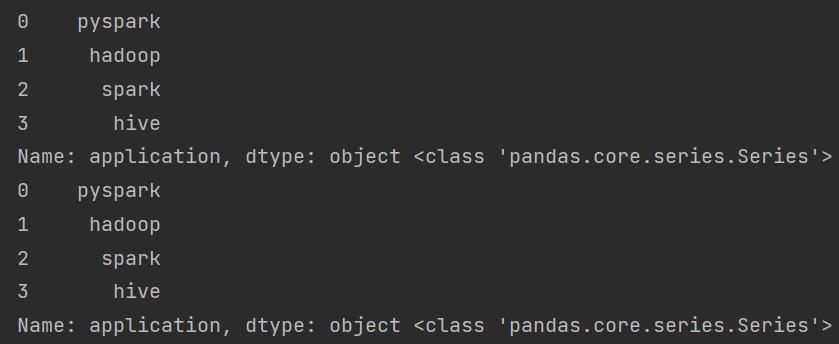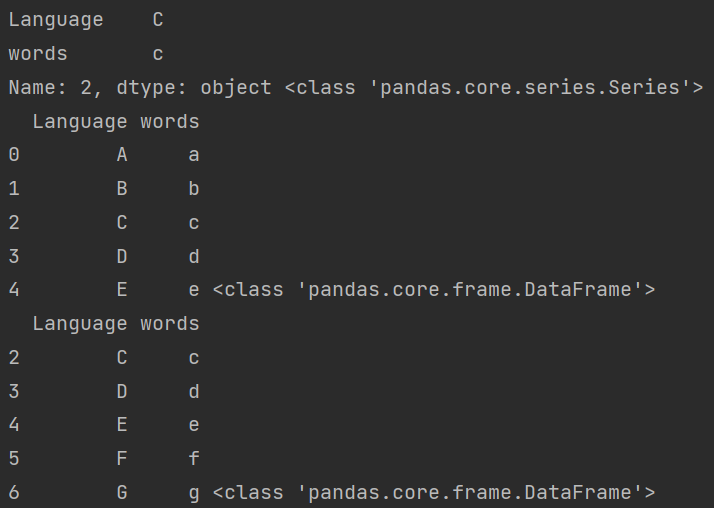### 根据具体条件获取数据

``````# 创建一个学生DataFrame
data6 = {
"name": ["张同学", "李同学", "王同学"],
"age": [17, 19, 16]
}
df6 = pd.DataFrame(data=data6)
print(df6)
# 获取年龄小于18的结果
print("----获取年龄小于18的结果----")
print(df6["age"] < 18)
# 进一步获取年龄小于18的同学的信息
print("----进一步获取年龄小于18的同学的信息----")
print(df6[df6["age"] < 18])
``````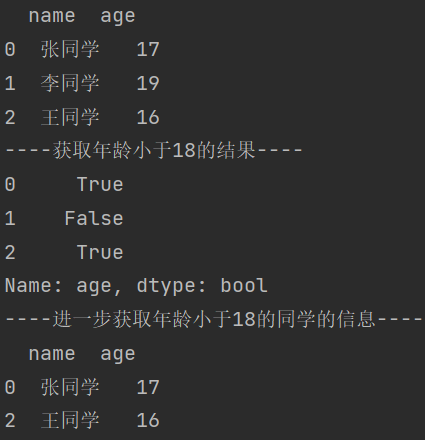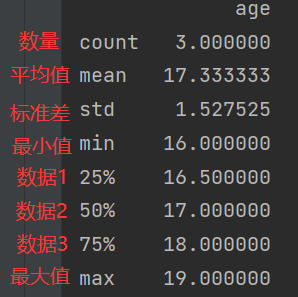## 文件操作

``````# 读取文件
df7 = pd.read_csv("D:\PyCharmProjects\day47\data\students.txt", names=["id", "name", "age", "gender", "clazz"])
print(df7)
``````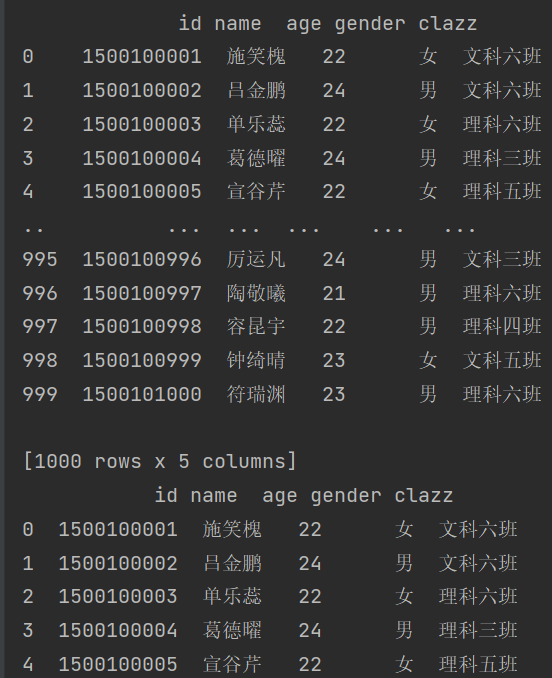path :表示文件系统位置、URL、文件型对象的字符串
sep/delimiter:用于对行中个字段进行拆分的字符序列或正则表达式
na_values:一组用于替换NA的值
nrows:需要读取的行数(从文件开始处算起)
verbose:打印各种解析器信息，比如"非数值列中缺失值的数量"
encoding:用于unicode的文本格式编码。例如，"utf-8"表示用UTF-8 编码的文本

# 使用jupyter notebook完成文件相关操作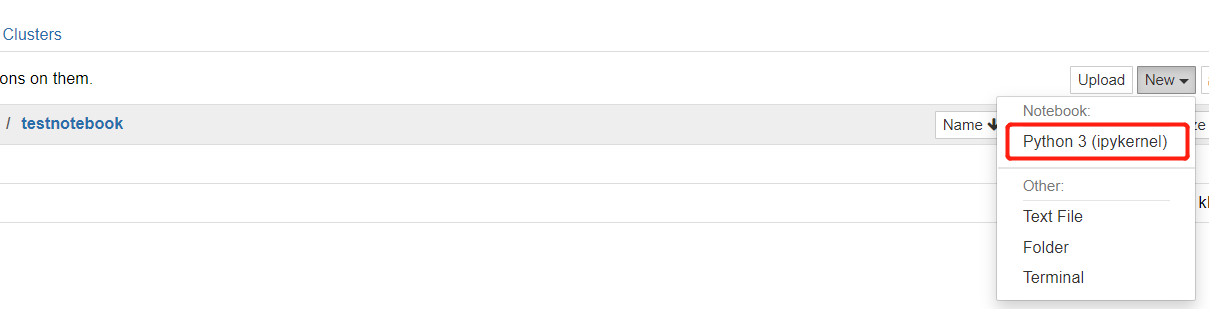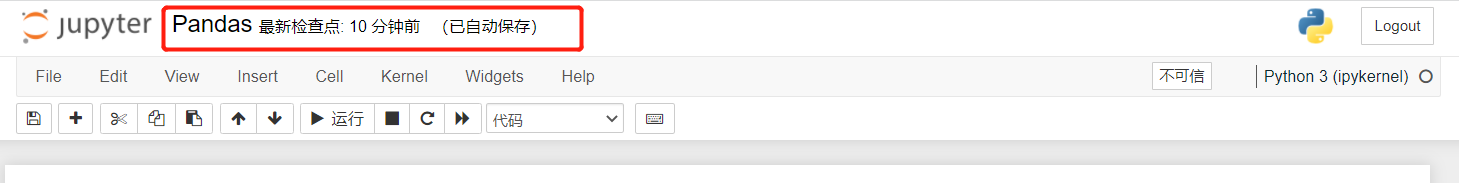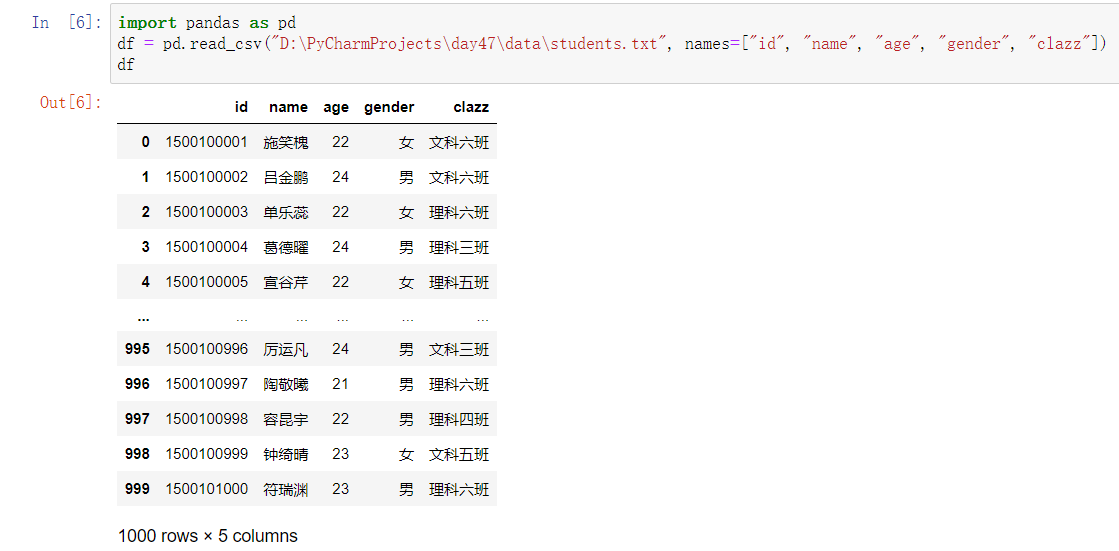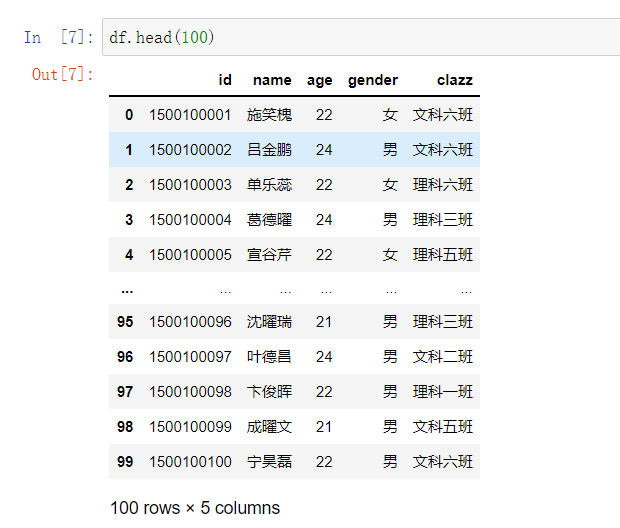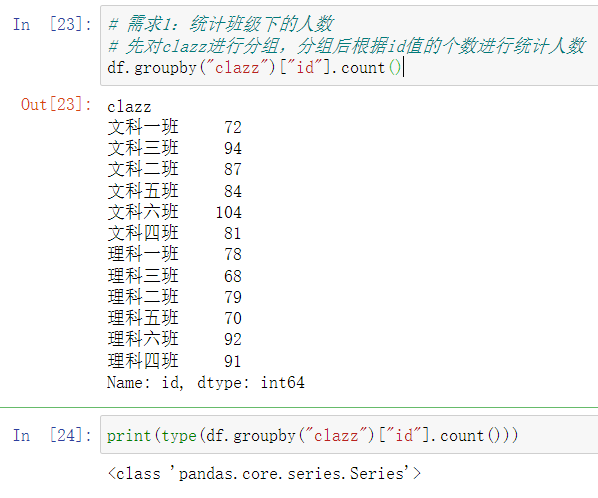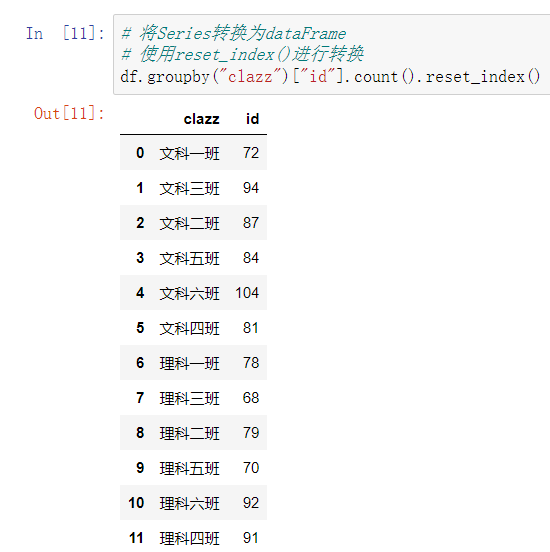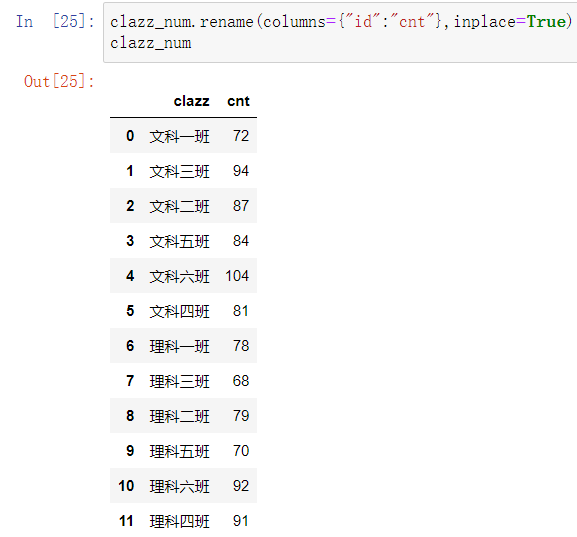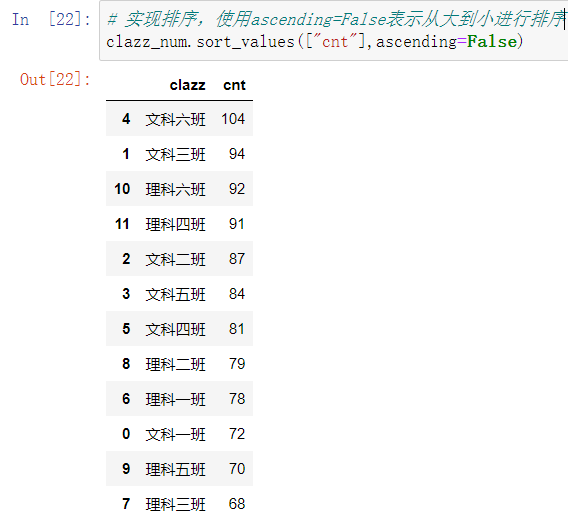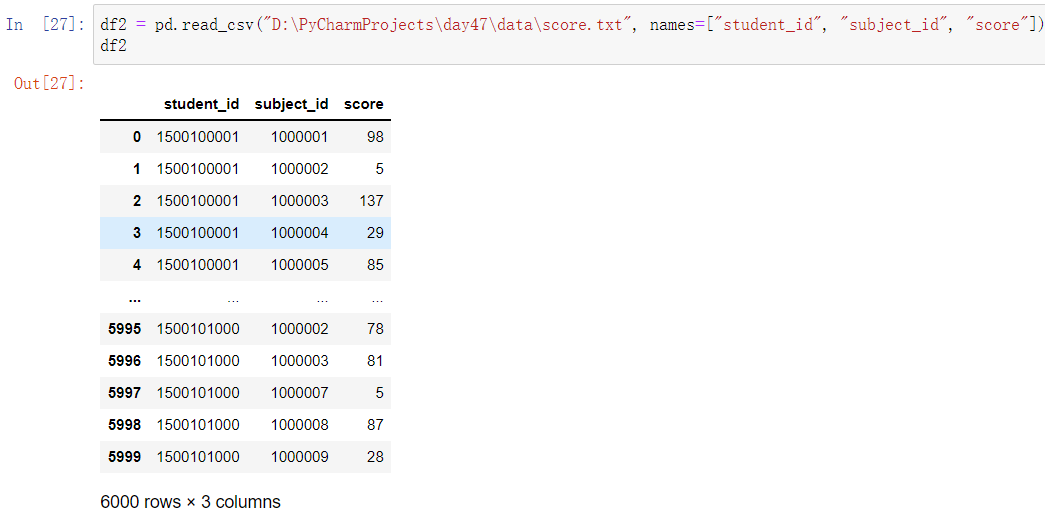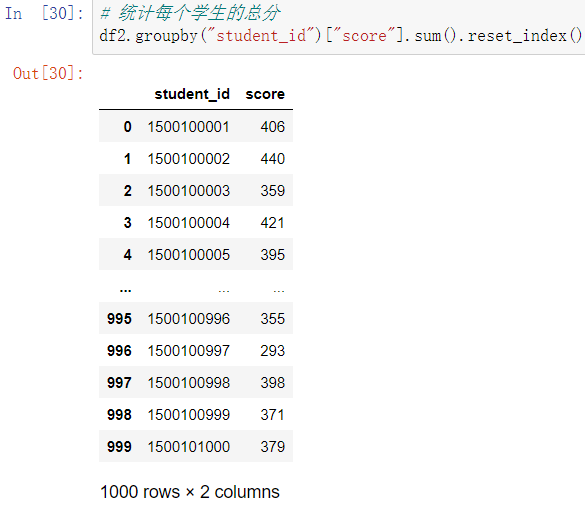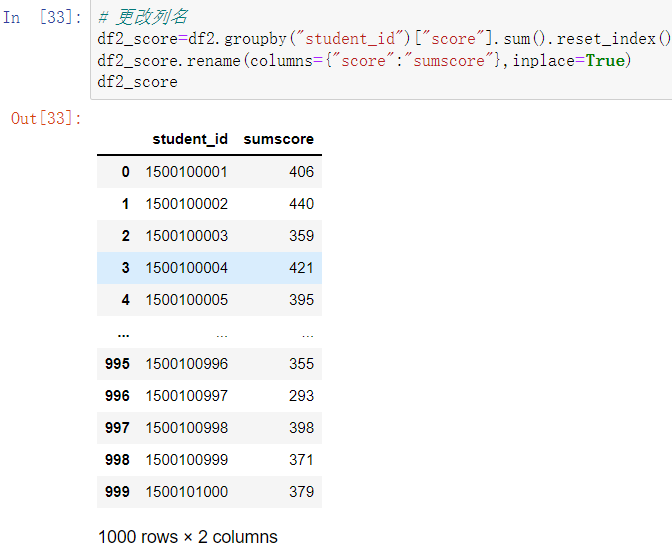left_on表示左表中用来关联的列名称

right_on表示右表中用来关联的列名称

how表示用来关联的方式，类似于sql中连表联查中的左联右联和内联，这其中的关联方式有很多种：left，right，outer，inner，cross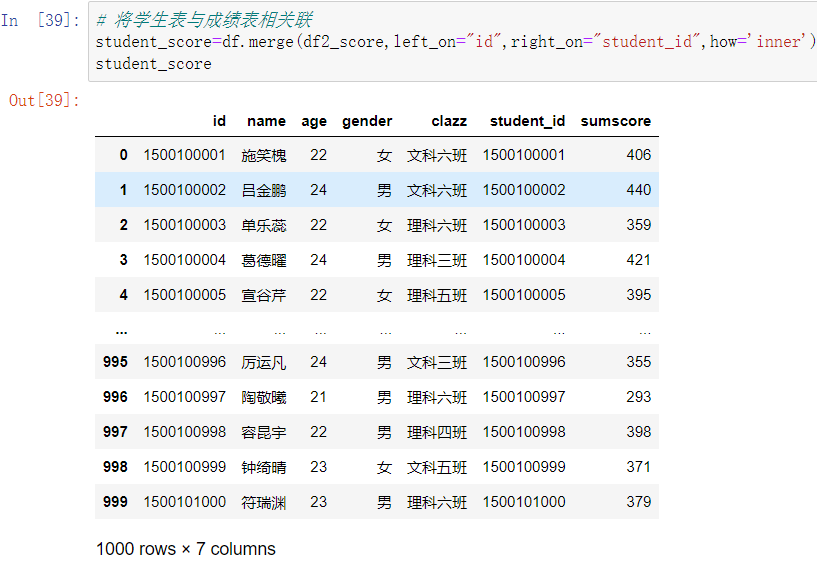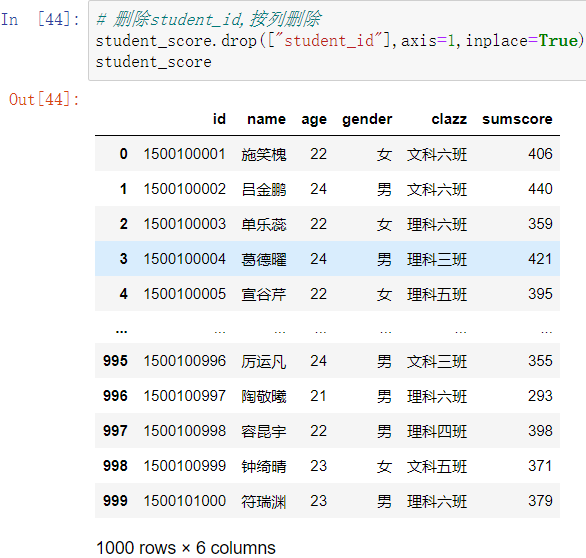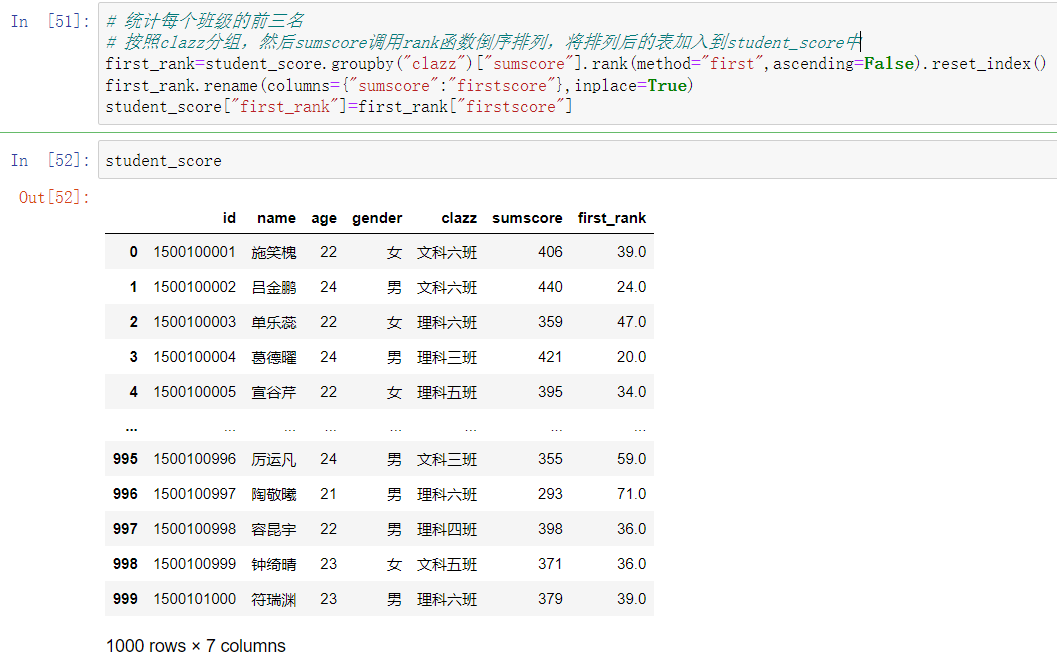Original: https://blog.csdn.net/m0_64350718/article/details/123780035
Author: Tian-Ys
Title: Day47 Pandas数据分析

# ModuleNotFoundError: No module named 'tensorflow'

`ModuleNotFoundError: No module named 'tensorflow'&#xFF1A;`

# ; 最直接的解决方案：

.

[En]

If you know the environment you are configuring and firmly believe that the installation is correct, follow the steps provided in the next section.

# 如果是在 Jupyter Notebook 里报错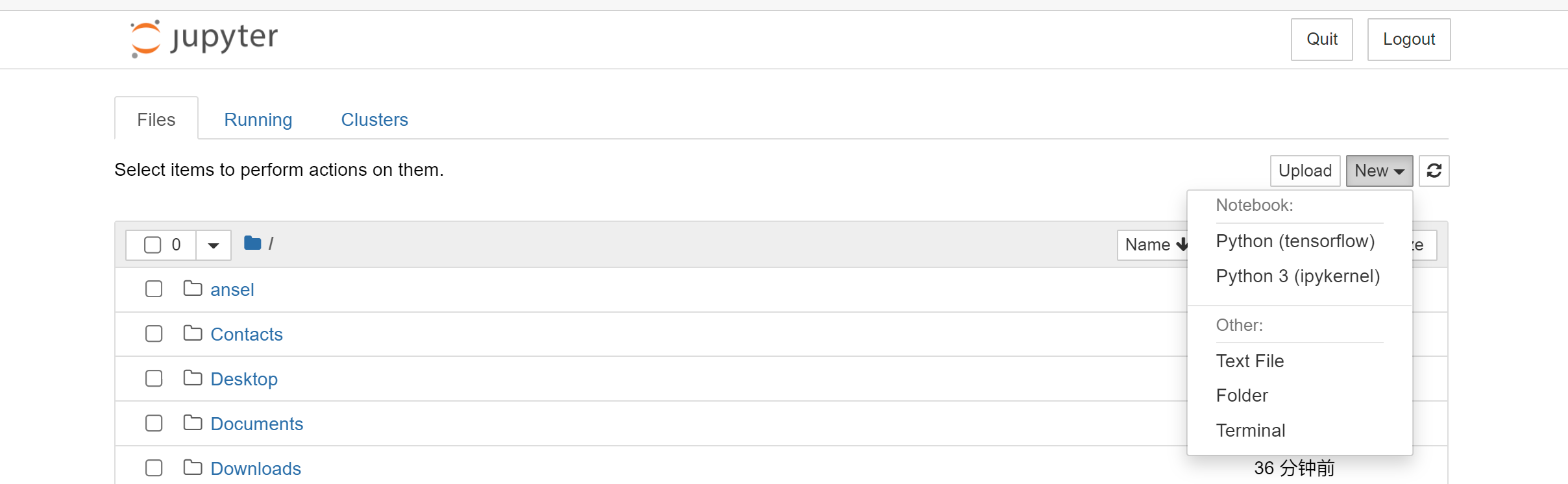# ; 导入环境到 Jupyter Notebook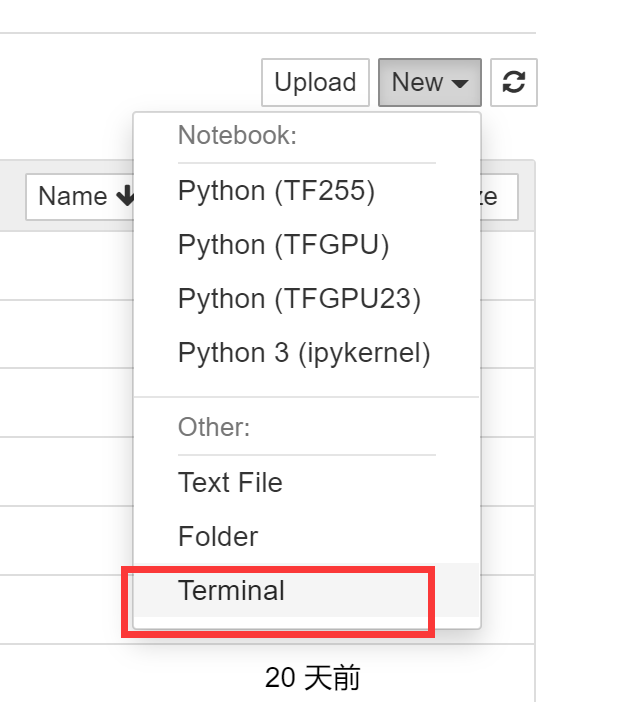``````conda env list
``````

``````python -m ipykernel install --user --name **环境名称** --display-name "Python (环境名称)"
``````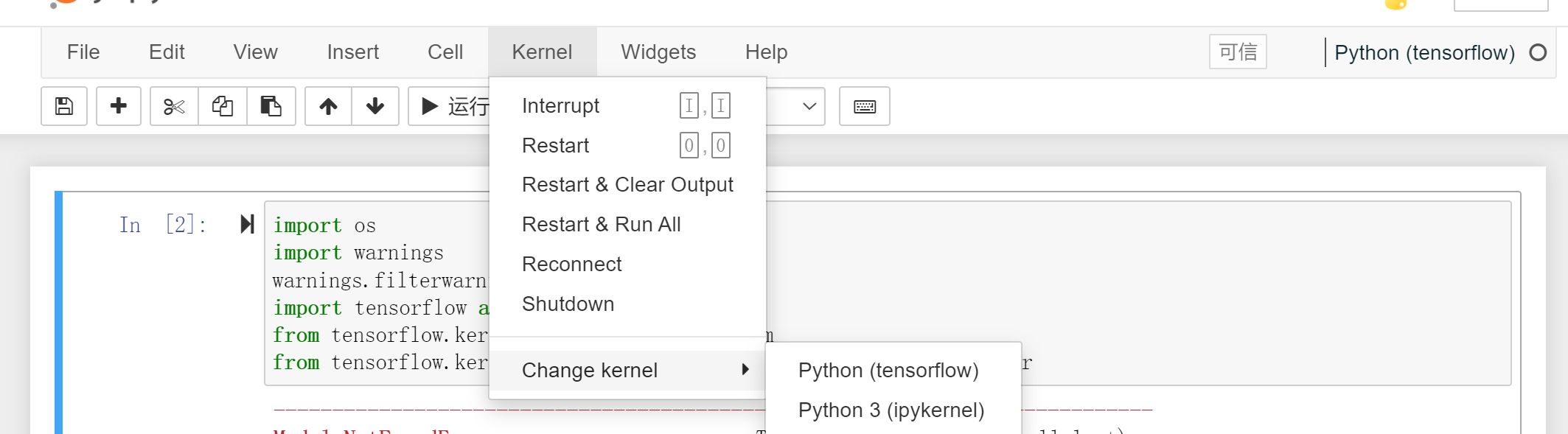# 还有一种比较小可能性是需要更新 Tensorflow 环境

``````conda env list
``````

``````activate **环境名称**
``````

``````pip install --upgrade --ignore-installed tensorflow-gpu -i https://pypi.douban.com/simple
``````

``````pip install --upgrade --ignore-installed tensorflow -i https://pypi.douban.com/simple
``````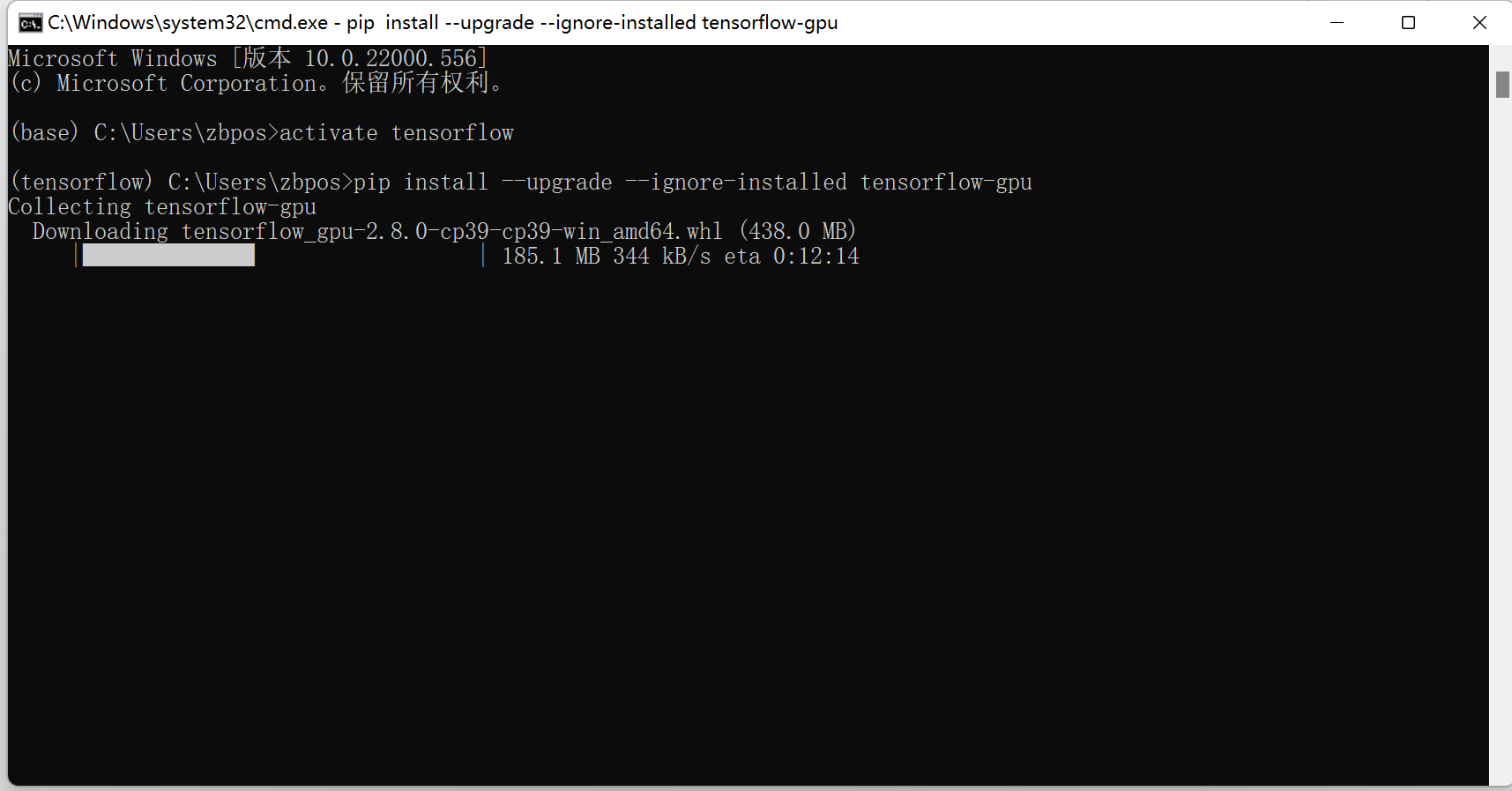``````阿里云
http://mirrors.aliyun.com/pypi/simple/

https://pypi.mirrors.ustc.edu.cn/simple/

http://pypi.douban.com/simple/

https://pypi.tuna.tsinghua.edu.cn/simple/

http://pypi.mirrors.ustc.edu.cn/simple/
``````

[En]

If there is still a red error report, simply and rudely delete this environment! It is not necessary to correct the error report, which is too time-consuming!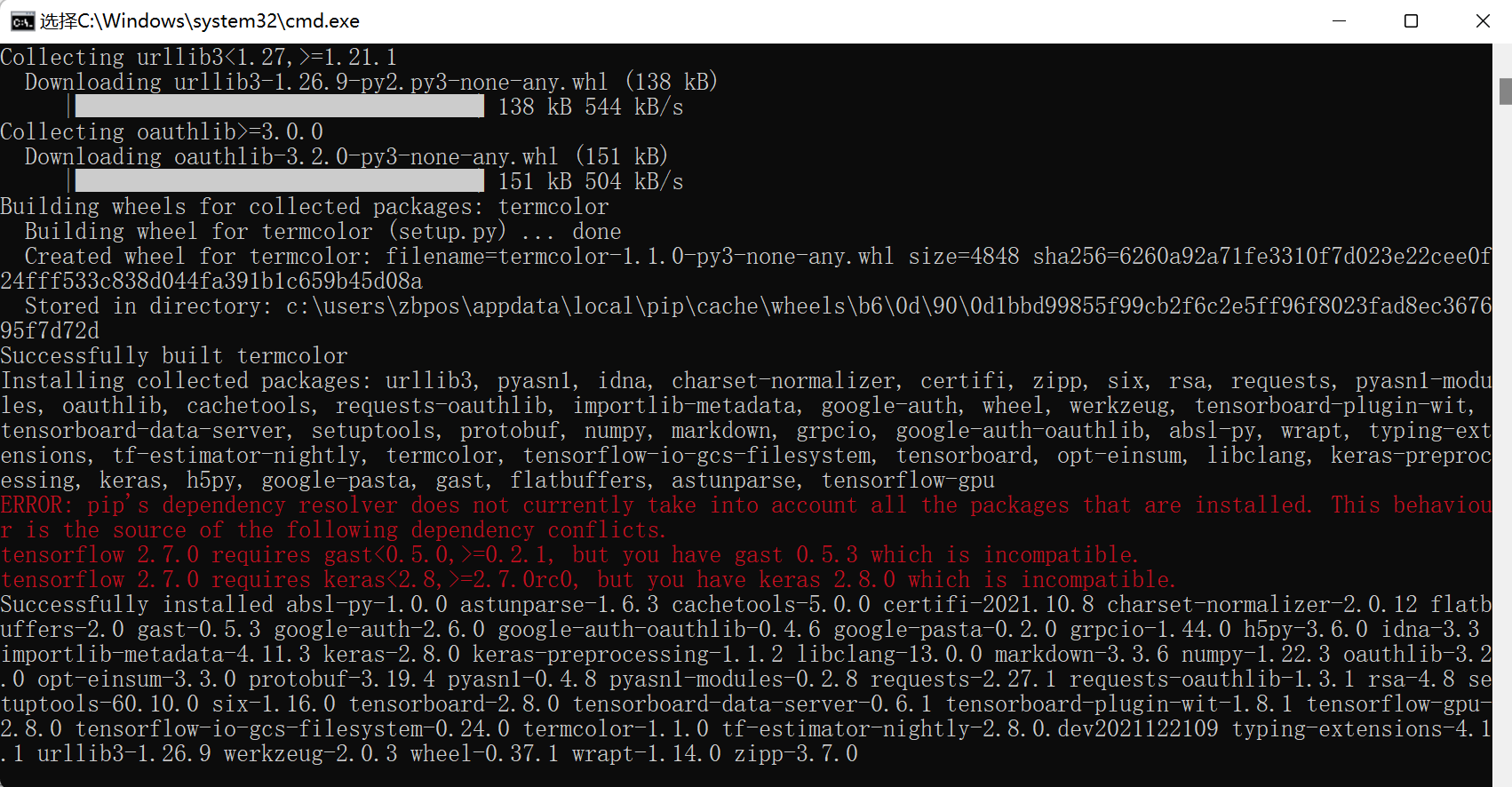Original: https://blog.csdn.net/weixin_44161490/article/details/123560760
Author: CV刘昊然
Title: ModuleNotFoundError: No module named ‘tensorflow‘

## tensorflow和numpy库中tensordot详解

tensordot函数的理解 函数的定义： axes =0 * 一维变量的计算 二维变量的计算 axes=1 * 一维向量的计算 二维向量的计算 三维向量的计算 axes=2 * 二维向量的计算 三维...